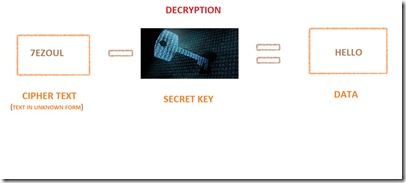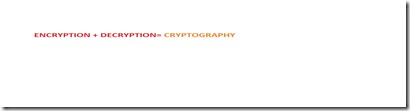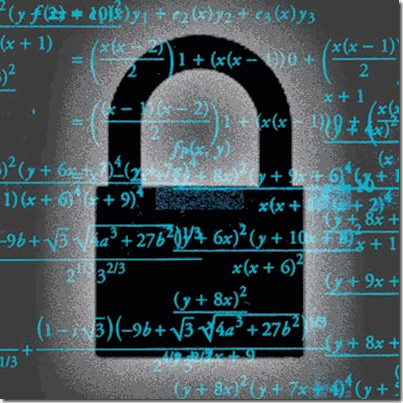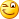# What is CRYPTOGRAPHY

Cryptography is a term used in Network Security. It is the process of converting plain text into unreadable format called as cipher text. The conversion of plain text into cipher text is called as Encryption and the process of converting cipher text into plain text is called as Decryption. The combined process of encryption and decryption is called as Cryptography.#### wHY WE NEED CRYPTOGRAPHY?We are using the internet for our each and every work. The work may be sending mail , important and confidential news , so we are in the situation to keep the data safely. So the cryptography is the technique used by the peoples who need to securely protect their data. (The process of cryptography is explained above)

Let me explain how this technique works.

There are two basic building blocks in encryption techniques are substitution and transposition.

1.Caesar cipher

2.Mono alphabetic cipher

3.Play fair cipher

4.Hill cipher

5.polyalphabetic cipher

are the types of algorithm used in substitution technique.

Now i am going to explain the concept using

Caesar cipher

this method is the basic of cryptography.

The steps of algorithm are:

1.Assign alphabets a to z with 0 to 25

2.Now take a plain text for example HELLO

3.We have to apply the algorithm

C=(P+K)MOD26

where,

C=cipher text

P= value of plaintext(i.e we assign numbers to alphabets )

K=secret key

Let the secret key be 3

now lets find the cipher text of the word HELLO

assigning numbers to alphabets

a  b  c  d  e  f  g  h  i   j   k    l   m   n    o   p    q

0  1  2  3  4  5  6  7  8  9  10 11 12  13  14  15  16

r     s    t    u    v    w   x    y    z

17  18  19  20  21  22  23  24  25

Now assign value for the word HELLO…

H=7

E=4

L=11

L=11

O=14

Just apply the values in the formula and find the corresponding alphabet to the corresponding values.

let we assume that KEY k= 3

C=(H+3)MOD26

C=(7+3)MOD26

C=10mod26

C=10

10=K

thus the letter H is transformed into the letter K, by assigning the values in the formula for the other letter the letters are transformed into

H=K

E=H

L=O

L=O

O=R

Thus the data HELLO is transformed into the cipher text

KHOOR.

Now we have successfully encrypted the data.

Let we decrypt the encrypted data …

It’s so simple to find the plain text from the cipher text use the algorithm

P=(C-K)MOD26

Let decrypt the first cipher K

P=(K-3)mod26

P=(10-3)mod26

P=7mod26

P=7

where, 7 is the  value of the letter H.

Is it interesting right !!!You can use this technique to share messages between your boy friends and girl friends for fun you all need do is that have the assigned values in a fixed paper and fix a key apply the values of the plain text and the key in the algorithm then send to your friend , say the key value separately to your friend so that no one will understand what you have written until they know the key value.

I think this is enough now about CRYPTOGRAPHY. There are several techniques used in the field of cryptography. We are just now in the beginning basics of the cryptography.